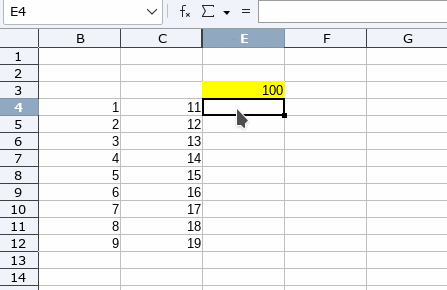# Fill cells with formula adapting references

How can I create a column in which each cell will calculate it’s SUM formula, then the cell below will calculate the ‘same’ formula having been incrementally altered by its location on the sheet?

cell e4 solves the formula =SUM(e3+b4-c4)
cell e5 solves the formula =SUM( e4+b5-c5)
and so on…

I want to use the fill series function but I can’t seem to make it work for this.

[Edit - Opaque] Changed title to short description and move old title to details

Hello

Preface

• Using function `SUM()` is superfluous in your case. Using `=E3+B4-C4` is sufficient.
• Using `Sheet -> Fill Cells -> Fill Series...` won’t support your goal.

Solution

Just do the following:

• Enter `=E3+B4-C4` into cell `E4`
• Select cell `E4`
• Drag down the formula using the black square in the lower right corner of cell `E4`

IllustrationIf the answer works for you, please consider to click the check mark () next to the answer. Thanks in advance …